# A simple program for an ANN to cover the Mnist dataset – VI – the math behind the „error back-propagation“

I continue with my article series on how to program a training algorithm for a multi-layer perceptron [MLP]. In the course of my last articles

we have already created code for the "Feed Forward Propagation" algorithm [FFPA] and two different cost functions - "Log Loss" and "MSE". In both cases we took care of a vectorized handling of multiple data records in mini-batches of training data.

Before we turn to the coding of the so called "error back-propagation" [EBP], I found it usefull to clarify the math behind behind this method for ANN/MLP-training. Understanding the basic principles of the gradient descent method for the optimization of MLP-weights is easy. But comprehending

• why and how gradient descent method leads to the back propagation of error terms
• and how we cover multiple training data records at the same time

is not - at least not in my opinion. So, I have discussed the required analysis and resulting algorithmic steps in detail in a PDF which you find attached to this article. I used a four layer MLP as an example for which I derived the partial derivatives of the "Log Loss" cost function for weights of the hidden layers in detail. I afterwards generalized the formalism. I hope the contents of the PDF will help beginners in the field of ML to understand what kind of matrix operations gradient descent leads to.

PDF on the math behind Error Back_Propagation

In the next article we shall encode the surprisingly compact algorithm for EBP. In the meantime I wish all readers Merry Christmas ...

Addendum 01.01.2020: Corrected a missing "-" for the cost function in the above PDF.

# Linux, OpenBlas and Numpy matrix multiplications – avoid using all processor cores

Recently, I tested the propagation methods of a small Python3/Numpy class for a multilayer perceptron [MLP]. I unexpectedly ran into a performance problem with OpenBlas.

The problem had to do with the required vectorized matrix operations for forward propagation - in my case through an artificial neural network [ANN] with 4 layers. In a first approach I used 784, 100, 50, 10 neurons in 4 consecutive layers of the MLP. The weight matrices had corresponding dimensions.

The performance problem was caused by extensive multi-threading; it showed a strong dependency on mini-batch sizes and on basic matrix dimensions related to the neuron numbers per layer:

• For the given relatively small number of neurons per layer and for mini-batch sizes above a critical value (N > 255) OpenBlas suddenly occupied all processor cores with a 100% work load. This had a disastrous impact on performance.
• For neuron numbers as 784, 300, 140, 10 OpenBlas used all processor cores with a 100% work load right from the beginning, i.e. even for small batch sizes. With a seemingly bad performance over all batch sizes - but decreasing somewhat with large batch sizes.

This problem has been discussed elsewhere with respect to the matrix dimensions relevant for the core multiplication and summation operations - i.e. the neuron numbers per layer. However, the vectorizing aspect of matrix multiplications is interesting, too:

One can imagine that splitting the operations for multiple independent test samples is in principle ideal for multi-threading. So, using as many processor cores as possible (in my case 8) does not look like a wrong decision of OpenBlas at first.

Then I noticed that for mini-batch sizes "N" below a certain number (N < 250) the system only seemed to use up to 3-4 cores; so there remained plenty of CPU capacity left for other tasks. Performance for N < 250 was better by at least a factor of 2 compared to a situation with an only slightly bigger batch size (N ≥ 260). I got the impression that OpenBLAS under certain conditions just decides to use as many threads as possible - which no good outcome.

In the last years I sometimes had to work with optimizing multi-threaded database operations on Linux systems. I often got the impression that you have to be careful and leave some CPU resources free for other tasks and to avoid heavy context switching. In addition bottlenecks appeared due to the concurrent access of may processes to the CPU cache. (RAM limitations were an additional factor; but this should not be the case for my Python program.) Furthermore, one should not forget that Python/Numpy experiments on Jupyter notebooks require additional resources to handle the web page output and page update on the browser. And Linux itself also requires some free resources.

So, I wanted to find out whether reducing the number of threads - or available cores - for Numpy and OpenBlas would be helpful in the sense of an overall gain in performance.

All data shown below were gathered on a desktop system with some background activity due to several open browsers, clementine and pulse-audio as active audio components, an open mail client (kontact), an open LXC container, open Eclipse with Pydev and open ssh connections. Program tests were performed with the help of Jupyter notebooks. Typical background CPU consumption looks like this on Ksysguard: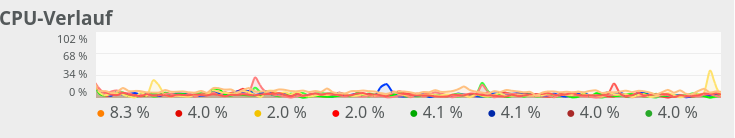Most of the consumption is due to audio. Small spikes on one CPU core due to the investigation of incoming mails were possible - but always below 20%.

# Basics

One of the core ingredients to get an ANN running are matrix operations. More precisely: multiplications of 2-dim Numpy matrices (weight matrices) with input vectors. The dimensions of the weight matrices reflect the node-numbers of consecutive ANN-layers. The dimension of the input vector depends on the node number of the lower of two neighbor layers.

However, we perform such matrix operations NOT sequentially sample for sample of a collection of training data - we do it vectorized for so called mini-batches consisting of between 50 and 600000 individual samples of training data. Instead of operating with a matrix on just one feature vector of one training sample we use matrix multiplications whereby the second matrix often comprises many vectors of data samples.

I have described such multiplications already in a previous blog article; see Numpy matrix multiplication for layers of simple feed forward ANNs.

In the most simple case of an MLP with e.g.

• an input layer of 784 nodes (suitable for the MNIST dataset),
• one hidden layer with 100 nodes,
• another hidden layer with 50 nodes
• and an output layer of 10 nodes (fitting again the MNIST dataset)

and "mini"-batches of different sizes (between 20 and 20000). An input vector to the first hidden layer has a dimension of 100, so the weight matrix creating this input vector from the "output" of the MLP's input layer has a shape of 784x100. Multiplication and summation in this case is done over the dimension covering 784 features. When we work with mini-batches we want to do these operations in parallel for as many elements of a mini-batch as possible.

All in all we have to perform 3 matrix operations

(784x100) matrix on (784)-vector, (100x50) matrix on (100)-vector, (50x10) matrix on (50) vector

on our example ANN with 4 layers. However, we collect the data for N mini-batch samples in an array. This leads to Numpy matrix multiplications of the kind

(784x100) matrix on an (784, N)-array, (100x50) matrix on an (100, N)-array, (50x10) matrix on an (50, N)-array.

Thus, we deal with matrix multiplications of two 2-dim matrices. Linear algebra libraries should optimize such operations for different kinds of processors.

# The reaction of OpenBlas to an MLP with 4 layers comprising 784, 100, 50, 10 nodes

On my Linux system Python/Numpy use the openblas-library. This is confirmed by the output of command "np.__config__.show()":

```openblas_info:
libraries = ['openblas', 'openblas']
library_dirs = ['/usr/local/lib']
language = c
define_macros = [('HAVE_CBLAS', None)]
blas_opt_info:
libraries = ['openblas', 'openblas']
library_dirs = ['/usr/local/lib']
language = c
define_macros = [('HAVE_CBLAS', None)]
openblas_lapack_info:
libraries = ['openblas', 'openblas']
library_dirs = ['/usr/local/lib']
language = c
define_macros = [('HAVE_CBLAS', None)]
lapack_opt_info:
libraries = ['openblas', 'openblas']
library_dirs = ['/usr/local/lib']
language = c
define_macros = [('HAVE_CBLAS', None)]
```

and by

```(ml1) myself@mytux:/projekte/GIT/ai/ml1/lib64/python3.6/site-packages/numpy/core> ldd  _multiarray_umath.cpython-36m-x86_64-linux-gnu.so
linux-vdso.so.1 (0x00007ffe8bddf000)
libopenblasp-r0-2ecf47d5.3.7.dev.so => /projekte/GIT/ai/ml1/lib/python3.6/site-packages/numpy/core/./../.libs/libopenblasp-r0-2ecf47d5.3.7.dev.so (0x00007fdd9d15f000)
libm.so.6 => /lib64/libm.so.6 (0x00007fdd9ce27000)
libc.so.6 => /lib64/libc.so.6 (0x00007fdd9c84f000)
/lib64/ld-linux-x86-64.so.2 (0x00007fdd9f4e8000)
libgfortran-ed201abd.so.3.0.0 => /projekte/GIT/ai/ml1/lib/python3.6/site-packages/numpy/core/./../.libs/libgfortran-ed201abd.so.3.0.0 (0x00007fdd9c555000)

```

In all tests discussed below I performed a series of calculations for different batch sizes

N = 50, 100, 200, 250, 260, 500, 2000, 10000, 20000

and repeated the full forward propagation 30 times (corresponding to 30 epochs in a full training series - but here without cost calculation and weight adjustment. I just did forward propagation.)

In a first experiment, I did not artificially limit the number of cores to be used. Measured response times in seconds are indicated in the following plot:

Runtime for a free number of cores to use and different batch-sizes N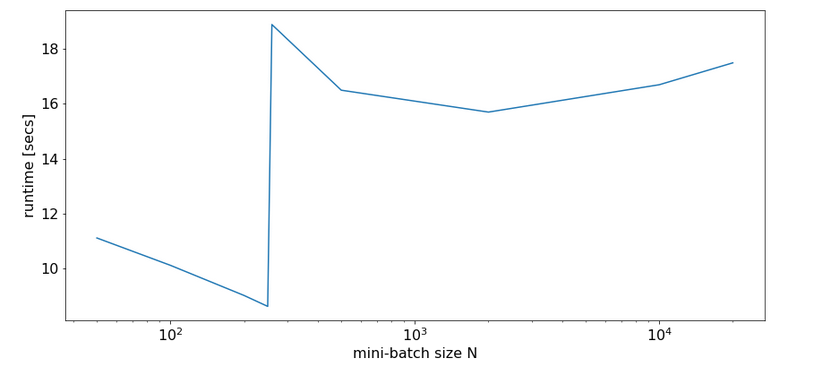We see that something dramatic happens between a batch size of 250 and 260. Below you see the plots for CPU core consumption for N=50, N=200, N=250, N=260 and N=2000.

The plots indicate that everything goes well up to N=250. Up to this point around 4 cores are used - leaving 4 cores relatively free. After N=260 OpenBlas decides to use all 8 cores with a load of 100% - and performance suffers by more than a factor of 2.

This result support the idea to look for an optimum of the number of cores "C" to use.

# The reaction of OpenBlas to an MLP with layers comprising 784, 300, 140, 10 nodes

For a MLP with neuron numbers (784, 300, 140, 10) I got the red curve for response time in the plot below. The second curve shows what performance is possible with just using 4 cores: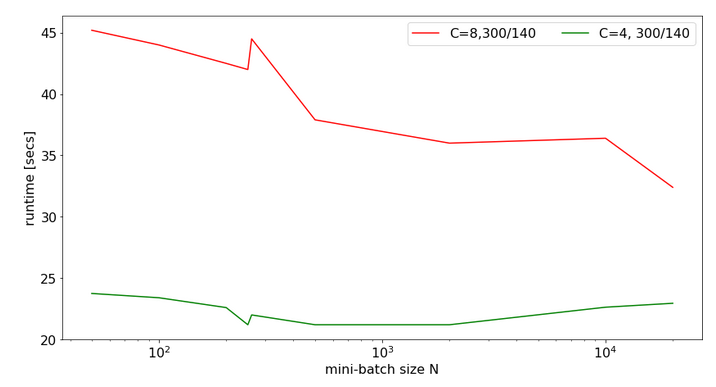Note the significantly higher response times. We also see again that something strange happens at the change of the batch-size from 250 to 260.

The 100% CPU consumption even for a batch-size of only 50 is shown below: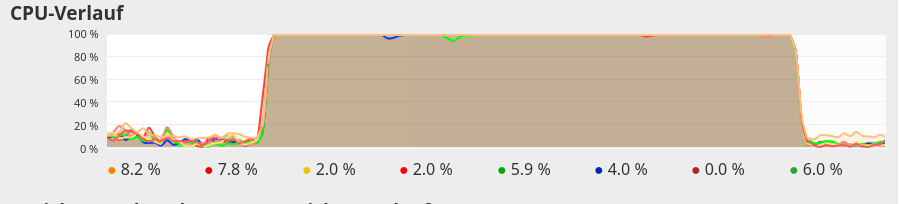Though different from the first test case also these plots indicate that - somewhat paradoxically - reducing the number of CPU cores available to OpenBlas could have a performance enhancing effect.

# Limiting the number of available cores to OpenBlas

A bit of Internet research shows that one can limit the number of cores to use by OpenBlas e.g. via an environment variable for the shell, in which we start a Jupyter notebook. The relevant command to limit the number of cores "C" to 3 is :

Below you find plots for the response times required for the batch sizes N listed above and core numbers of

C=1, C=2, C=3, C=4, C=5, C=6, C=7, C=8 :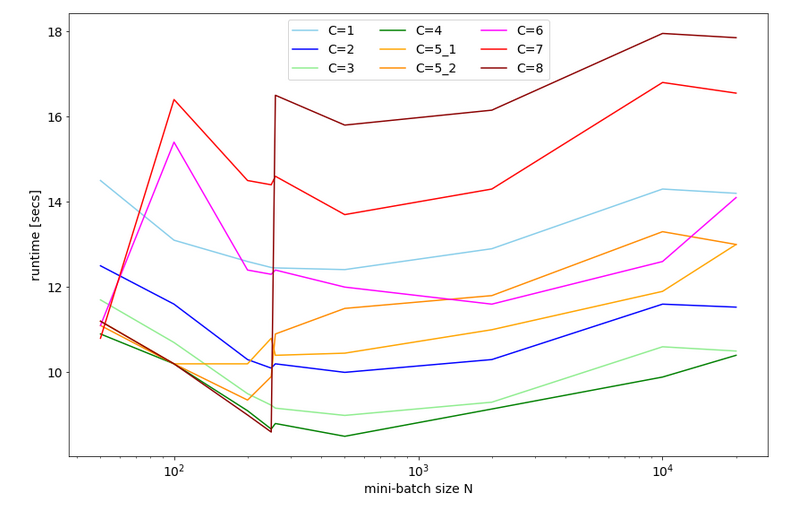For C=5 I did 2 different runs; the different results for C=5 show that the system reacts rather sensitively. It changes its behavior for larger core number drastically.

We also find an overall minimum of the response time:
The overall optimum occurs for 400 < N < 500 for C=1, 2, 3, 4 - with the minimum region being broadest for C=3. The absolute minimum is reached on my CPU for C=4.

We understand from the plots above that the number of cores to use become hyper-parameters for the tuning of the performance of ANNs - at least as long as a standard multicore-CPU is used.

# CPU-consumption

CPU consumption for N=50 and C=2 looks like: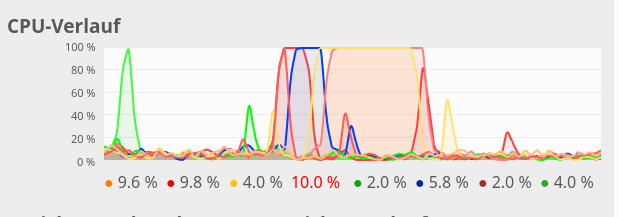For comparison see the CPU consumption for N=20000 and C=4: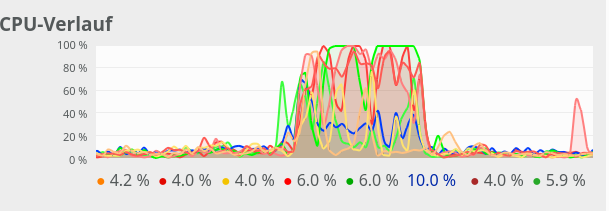CPU consumption for N=20000 and C=6: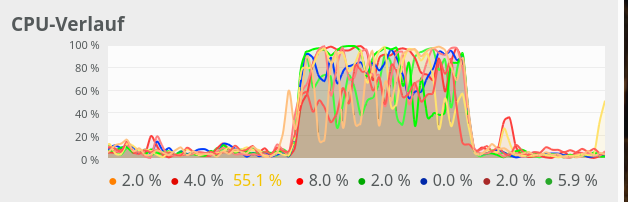We see that between C=5 and C=6 CPU resources get heavily consumed; there are almost no reserves left in the Linux system for C ≥ 6.

# Dependency on the size of the weight-matrices and the node numbers

For a full view on the situation I also looked at the response time variation with node numbers for a given number of CPU cores.

For C=4 and node number cases

• 784, 300, 140, 10
• 784, 200, 100, 10
• 784, 100, 50, 10
• 784, 50, 20, 10

I got the following results: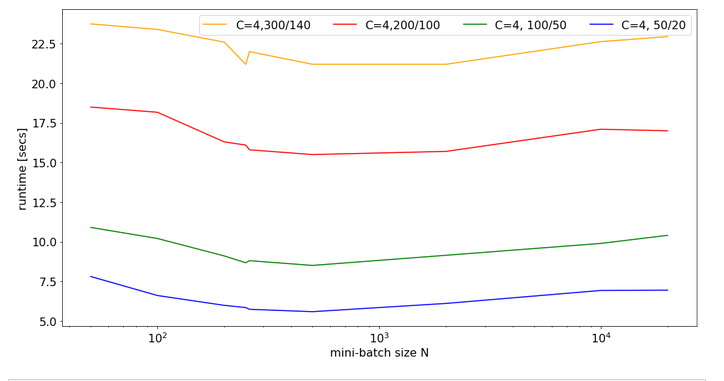There is some broad variation with the weight-matrix size; the bigger the weight-matrix the longer the calculation time. This is, of course, to be expected. Note that the variation with the batch-size number is relatively smooth - with an optimum around 400.

Now, look at the same plot for C=6: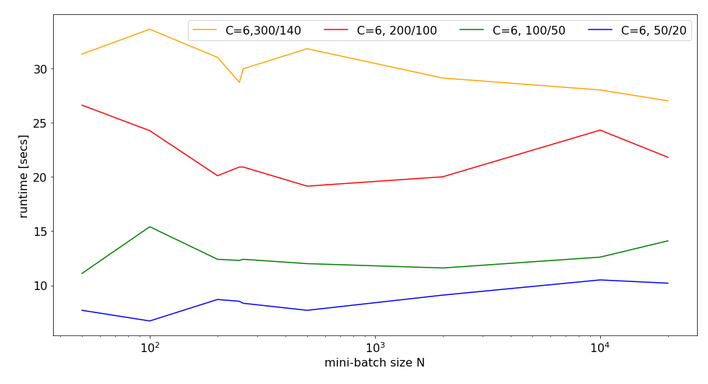Note that the response time is significantly bigger in all cases compared to the previous situation with C=4. In cases of a large matrix by around 36% for N=2000. Also the variation with batch-size is more pronounced.

Still, even with 6 cores you do not get factors between 1.4 and 2.0 as compared to the case of C=8 (see above)!

# Conclusion

As I do not know what the authors of OpenBlas are doing exactly, I refrain from technically understanding and interpreting the causes of the data shown above.

However, some consequences seem to be clear:

• It is a bad idea to provide all CPU cores to OpenBlas - which unfortunately is the default.
• The data above indicate that using only 4 out of 8 core threads is reasonable to get an optimum performance for vectorized matrix multiplications on my CPU.
• Not leaving at least 2 CPU cores free for other tasks is punished by significant performance losses.
• When leaving the decision of how many cores to use to OpenBlas a critical batch-size may exist for which the performance suddenly breaks down due to heavy multi-threading.

Whenever you deal with ANN or MLP simulations on a standard CPU (not GPU!) you should absolutely care about how many cores and related threads you want to offer to OpenBlas. As far as I understood from some Internet articles the number of cores to be used can be not only be controlled by Linux (shell) environment variables but also by os-commands in a Python program. You should perform tests to find optimum values for your CPU.

stackoverflow: numpy-suddenly-uses-all-cpus

stackoverflow: run-openblas-on-multicore

stackoverflow: multiprocessing-pool-makes-numpy-matrix-multiplication-slower

scicomp: why-isnt-my-matrix-vector-multiplication-scaling/1729

Setting the number of threads via Python
stackoverflow:

# Numpy matrix multiplication for layers of simple feed forward ANNs

I move a small step forward on my trials as a beginner in machine learning. This is once again going to be an article from which experts on "Artificial Neural networks" [ANNs] will learn nothing new. But some of my readers may also be newbies both to Python, to numpy and to ANNs. For them this article may be helpful.

I shall have a brief look at some matrix (or array) operations which constitute important parts of the information propagation on ANNs. How would such operations look like in Python with Numpy? One should have a clear understanding of the so called "dot"-operation between multidimensional arrays in this context. Such operations can be performed highly optimized on GPUs - and whole sets of data samples can be handled in form of vectorized code instructions. I will try to explain this in form of matrix terms required to simulate information transport between some layers of a very simple network.

# Propagation operations on ANNs

Basic Artificial Neural Networks consist of layers with neurons. Each layer has a defined number of neurons. Each neuron is connected with all neurons of the next layer (receiving neurons). The output "O(k, L)" of a neuron N(k,L) of Layer "L" to a neuron N(m,L+1) is multiplied by some "weight" W[(m, L+1),(k, L),].

The contributions of all neurons on layer "L" to N(m, L+1) are summed up. The result is then transformed by some local "activation" function and afterwards presented by N(m, L+1) as output which shall be transported to the neurons of layer L+2. The succession of such operations is called "propagation".

At the core of propagation we, therefore, find operations which we can express in the following form :

Input vector at N(m, L+1) : I(m, L+1) = SUM(over_all_k) [ W[(m, L+1), (k, L)] * O(k, L) ]
Output vector at N(m, L+1) : O(m, L+1) = f_act( I(m, L+1) )

To make things simpler: Let us set f_act = 1. Then we get

O(m, L+1) = SUM(over all k) [ W[(m, L+1), (k,N)] * O(k, L) ]

The SUM is basically a matrix operations on vector O(k, L). If output vectors were always given by singular values in "k" rows (!) then we could write

O(L+1) = W(L, L+1) * O(L) )

# Batches and numerical optimization of the matrix operations for propagation

In the past I have programmed gas flow simulations in exploding stars in Fortran/C and simulated cascaded production networks for Supply Chains in PHP. There, you work with deep and complicated layer structures, too. Therefore I felt well prepared for writing a small program to set up a simple artificial neural network with some hidden layers.

Something, you will find in literature (e.g. in the book of S. Rashka, "Python machine Learning", 2016, PACKT Publishing] are remarks on batch based training. Rashka e.g. rightfully claims in his discussion of an example code that the use of mini-batches of input data sets is advantageous (see chapter 12, page 379 in the book mentioned above):

"Mini-batch learning has the advantage over online learning that we can make use of our vectorized implementations to improve computational efficiency."

I swallowed this remark first without much thinking. As a physicist I had used highly optimized libraries for Linear Algebra operation on supercomputers with vector registers already 35 years ago. Ok, numpy can perform a matrix operation on some vector very fast, especially on GPUs - so what?

But when I tried to follow the basic line of programming of Rashka for an ANN in Python, I suddenly found that I did not fully understand what the code was doing whilst handling batches of input data sets. The reason was that I had not at all grasped the full implications of the quoted remark. It indicated not just the acceleration of a matrix operation on one input vector; no, instead, it indicated that we could and should perform matrix operations in parallel on all input vectors given by a full series of many different data sets in a mini-batch collection.

So, we then want to perform an optimized matrix operation on another matrix of at least 2 dimensions. When you think about it, you will quickly understand that any kind of operation of one n-dim matrix on another matrix with more and different dimensions must be defined very precisely to avoid misunderstandings and miscalculations.

To make things simple:
Let us take a (3,5)-matrix A and a (10,3)-matrix B. What should a "dot"-like operation A * B mean in this case? Would it be suitable at all, regarding the matrix element arrangements in rows and columns?

I studied the numpy documentation on "numpy.dot(A, B)". See e.g. here. Ooops, not fully trivial: "If A is an N-D array and B is an M-D array (where M>=2), it is a sum product over the last axis of A and the second-to-last axis of B". The matrices must obviously be arranged in a suitable way ....

So, I decided to performing some simple matrix experiments to get a clear understanding. I assumed a fictitious ANN of three layers and some direct propagation between the layers. What does propagation mean in such a case, how is it expressed in matrix operation terms in Python and what does batch-handling mean in this context?

# Three simple layers and a batch of input data sets

The following graphics shows my simple network: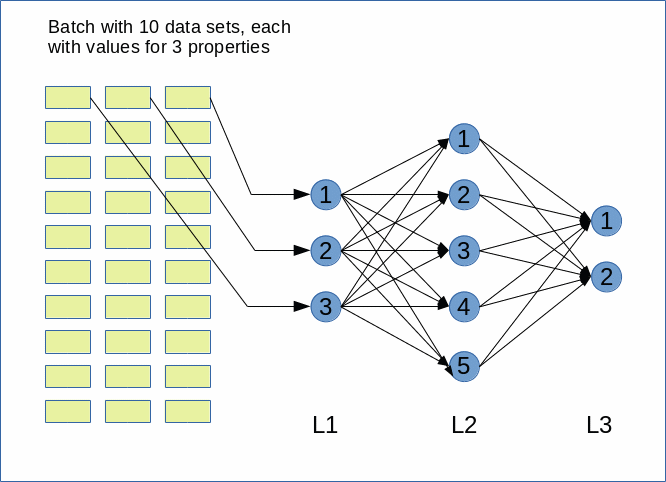Our batch has 10 data sets of test data for our ANN. Each data set describes 3 properties; thus the input layer "L1" has 3 nodes. Our "hidden" layer "L2" shall have 5 nodes and our output layer "L3" only 2 nodes.

# Weight matrices and propagation operations

Now, let us define some simple matrices with input vectors for transportation and weight-matrices for the layer-connections with Python. All activation functions shall in our simple example just perform a multiplication by 1.

The input to layer "L1" - i.e. a batch of 10 data sets - shall be given by a matrix of 10 lines (dimension 1) and 3 columns (dimension 2)

```import numpy as np
import scipy
import time

A1 = np.ones((10,3))
A1[:,1] *= 2
A1[:,2] *= 4
print("\nMatrix of input vectors to layer L1:\n")
print(A1)

print("\nMatrix of input vectors to layer 1:\n")
print(A1)

Matrix of input vectors to layer 1:

Matrix of input vectors to layer L1:

[[1. 2. 4.]
[1. 2. 4.]
[1. 2. 4.]
[1. 2. 4.]
[1. 2. 4.]
[1. 2. 4.]
[1. 2. 4.]
[1. 2. 4.]
[1. 2. 4.]
[1. 2. 4.]]
```

We now define the weight matrix "W1" for transport between layer L1 and layer L2 as:

```W1= np.random.randint(1, 10, 5*3)
W1 = w1.reshape(5,3)
print("\nMatrix of weights W1(L1, L2) :\n")
print(W1)

Matrix of weights W1(L1, L2) :

[[3 8 5]
[4 9 7]
[4 1 3]
[8 8 4]
[3 1 9]]

```

We set the first dimension to the number of nodes in the target layer (L2) and the second dimension to the number of nodes in the lower preceding layer (here: L1). Expressed in the shape notation for an array "(dimension 1, dimension 2, ...) ":

Shape of W : (dim 1, dim 2) = (node number of target layer, node number of preceding layer)

If we now tried a numpy "dot"-operation for our matrices

```A2 = np.dot(W1, A1)
```

we would get an error:

ValueError: shapes (5,3) and (10,3) not aligned: 3 (dim 1) != 10 (dim 0)

Well, this is actually consistent with the numpy documentation: The last dimension of "W1" should be consistent with the second to last (=first) dimension of "strong>A1".

What we need to do here is to transpose the matrix of our input vectors for our multiple data sets:

```A1 = np.transpose(A1)
print("\nMatrix of output vectors of layer 1 to layer 2:\n")
print(A1)

Matrix of output vectors of layer 1 to layer 2:

[[1. 1. 1. 1. 1. 1. 1. 1. 1. 1.]
[2. 2. 2. 2. 2. 2. 2. 2. 2. 2.]
[4. 4. 4. 4. 4. 4. 4. 4. 4. 4.]]

```

Then

```A2 = np.dot(W1, A1)
print("\n\nA2:\n")
print(A2)

A2:

[[39. 39. 39. 39. 39. 39. 39. 39. 39. 39.]
[50. 50. 50. 50. 50. 50. 50. 50. 50. 50.]
[18. 18. 18. 18. 18. 18. 18. 18. 18. 18.]
[40. 40. 40. 40. 40. 40. 40. 40. 40. 40.]
[41. 41. 41. 41. 41. 41. 41. 41. 41. 41.]]

```

Now, we need a weight matrix W2 between layer L1 and layer L2:

```W2= np.random.randint(1, 3, 2*5)
W2 = W2.reshape(2,5)
print("\n\nW2:\n")
print(W2)

W2:
[[2 2 1 2 2]
[2 2 2 2 1]]

```

We followed the same policy - and used the number of nodes in target layer L2 as the first dimension of the weight array.

Now, do we have to to anything with array A2, if we want to use it as an input for the next matrix operation ?

```A3 = np.dot(W2, A2)
```

No, we do not need to change anything! "A2" has the required form, already. The second to last dimension in "A2" is 5 in our example - as is the last dimension in "W2"!

So, the following will work directly :

```A3 = np.dot(W2, A2)
print("\n\nA3:\n")
print(A3)

A3:

[[358. 358. 358. 358. 358. 358. 358. 358. 358. 358.]
[335. 335. 335. 335. 335. 335. 335. 335. 335. 335.]]

```

You can try out our complete "propagation" code in a Jupyter notebook cell.

```import numpy as np
import scipy
import time

A1 = np.ones((10,3))
A1[:,1] *= 2
A1[:,2] *= 4
print("\nMatrix of input vectors to layer L1:\n")
print(A1)

W1= np.random.randint(1, 10, 5*3)
W1 = W1.reshape(5,3)
print("\nMatrix of weights W1(L1, L2) :\n")
print(W1)

A1 = np.transpose(A1)
print("\nMatrix of output vectors of layer L1 to layer L2:\n")
print(A1)

A2 = np.dot(W1, A1)
print("\n\nA2:\n")
print(A2)

W2= np.random.randint(1, 3, 2*5)
W2 = W2.reshape(2,5)
print("\n\nW2:\n")
print(W2)

A3 = np.dot(W2, A2)
print("\n\nA3:\n")
print(A3)
```

# Conclusion

The first thing we learned is that matrix operations on simple vectors can be extended to a set of vectors, i.e. a matrix. In the case of ANNs one dimension of such an (numpy) array would cover the number of data sets of a typical input mini-batch. The other dimension would cover the "properties" of the input data.

The second thing we saw is that the input matrix to an ANN often must be transposed to work together with weight matrices, if such matrices follow the policy that the first dimension is given by the number of cells in the target layer and the second dimension by the number of nodes of the preceding layer.

The weighted input to the first target layer is then given by a matrix "dot"-operation on the transposed input matrix. The outcome is a matrix where the first dimension is defined by the number of nodes of the target layer and the second dimension the number of data sets. This has already the correct form for a further propagation to the next layer. Note that the application of an activation function to each of the matrix elements would not change the required arrangement of matrix data!

After the input layer we, therefore, can just continue to directly apply weight matrices to output matrices by a numpy-"dot"-operation. The weight matrix structure just should reflect our shape policy of

(dim1, dim2) = (node number of target layer, node number of preceding layer)

.

In the next articles we shall use these insights to build a Python class for the setup and training of a simple ANN with one or two hidden layers for the analysis of the famous MNIST dataset.